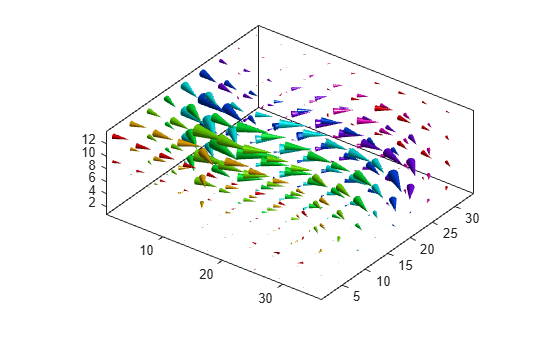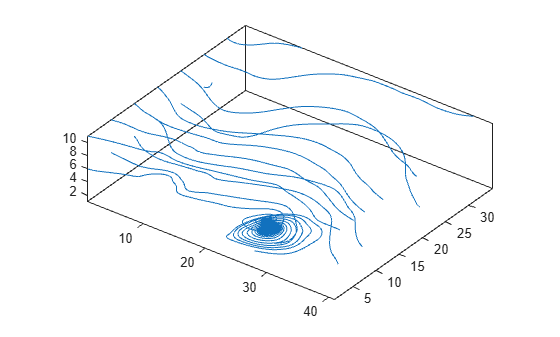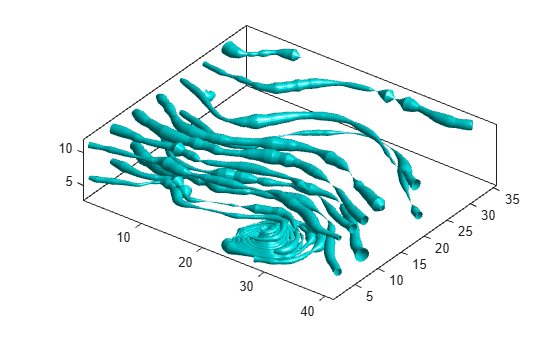Documentation

## Visualizing Volume Data

This example shows several methods for visualizing volume data in MATLAB®.

### Display Isosurface

An isosurface is a surface where all the points within a volume of space have a constant value. Use the `isosurface` function to generate the faces and vertices for the outside of the surface and the `isocaps` function to generate the faces and vertices for the end caps of the volume. Use the `patch` command to draw the volume and its end caps.

```load mri D % load data D = squeeze(D); % remove singleton dimension limits = [NaN NaN NaN NaN NaN 10]; [x, y, z, D] = subvolume(D, limits); % extract a subset of the volume data [fo,vo] = isosurface(x,y,z,D,5); % isosurface for the outside of the volume [fe,ve,ce] = isocaps(x,y,z,D,5); % isocaps for the end caps of the volume figure p1 = patch('Faces', fo, 'Vertices', vo); % draw the outside of the volume p1.FaceColor = 'red'; p1.EdgeColor = 'none'; p2 = patch('Faces', fe, 'Vertices', ve, ... % draw the end caps of the volume 'FaceVertexCData', ce); p2.FaceColor = 'interp'; p2.EdgeColor = 'none'; view(-40,24) daspect([1 1 0.3]) % set the axes aspect ratio colormap(gray(100)) box on camlight(40,40) % create two lights camlight(-20,-10) lighting gouraud```### Create Cone Plot

The `coneplot` command plots velocity vectors as cones at x, y, z points in a volume. The cones represent the magnitude and direction of the vector field at each point.

```cla % clear the current axes load wind u v w x y z % load data [m,n,p] = size(u); [Cx, Cy, Cz] = meshgrid(1:4:m,1:4:n,1:4:p); % calculate the location of the cones h = coneplot(u,v,w,Cx,Cy,Cz,y,4); % draw the cone plot set(h,'EdgeColor', 'none') axis tight equal view(37,32) box on colormap(hsv) light```### Plot Streamlines

The `streamline` function plots streamlines for a velocity vector at x, y, z points in a volume to illustrate the flow of a 3-D vector field.

```cla [m,n,p] = size(u); [Sx, Sy, Sz] = meshgrid(1,1:5:n,1:5:p); % calculate the starting points of the streamlines streamline(u,v,w,Sx,Sy,Sz) % draw the streamlines axis tight equal view(37,32) box on```### Plot Streamtubes

The `streamtube` function plots streamtubes for a velocity vector at x, y, z points in a volume. The width of the tube is proportional to the normalized divergence of the vector field at each point.

```cla [m,n,p] = size(u); [Sx, Sy, Sz] = meshgrid(1,1:5:n,1:5:p); % calculate the starting points of the streamlines h = streamtube(u,v,w,Sx,Sy,Sz); % draw the streamtubes and return an array of surfaces set(h, 'FaceColor', 'cyan') % use 'set' to change properties for an array of objects set(h, 'EdgeColor', 'none') axis tight equal view(37,32) box on light```### Combine Volume Visualizations

Combine volume visualization in a single plot to get a more comprehensive picture of a velocity field within a volume.

```cla spd = sqrt(u.*u + v.*v + w.*w); % wind speed at each point in the volume [fo,vo] = isosurface(x,y,z,spd,40); % isosurface for the outside of the volume [fe,ve,ce] = isocaps(x,y,z,spd,40); % isocaps for the end caps of the volume p1 = patch('Faces', fo, 'Vertices', vo); % draw the isosurface for the volume p1.FaceColor = 'red'; p1.EdgeColor = 'none'; p2 = patch('Faces', fe, 'Vertices', ve, ... % draw the end caps of the volume 'FaceVertexCData', ce); p2.FaceColor = 'interp'; p2.EdgeColor = 'none' ; [fc, vc] = isosurface(x, y, z, spd, 30); % isosurface for the cones [fc, vc] = reducepatch(fc, vc, 0.2); % reduce the number of faces and vertices h1 = coneplot(x,y,z,u,v,w,vc(:,1),vc(:,2),vc(:,3),3); % draw the coneplot h1.FaceColor = 'cyan'; h1.EdgeColor = 'none'; [sx, sy, sz] = meshgrid(80, 20:10:50, 0:5:15); % starting points for streamline h2 = streamline(x,y,z,u,v,w,sx,sy,sz); % draw the streamlines set(h2, 'Color', [.4 1 .4]) axis tight equal view(37,32) box on light```